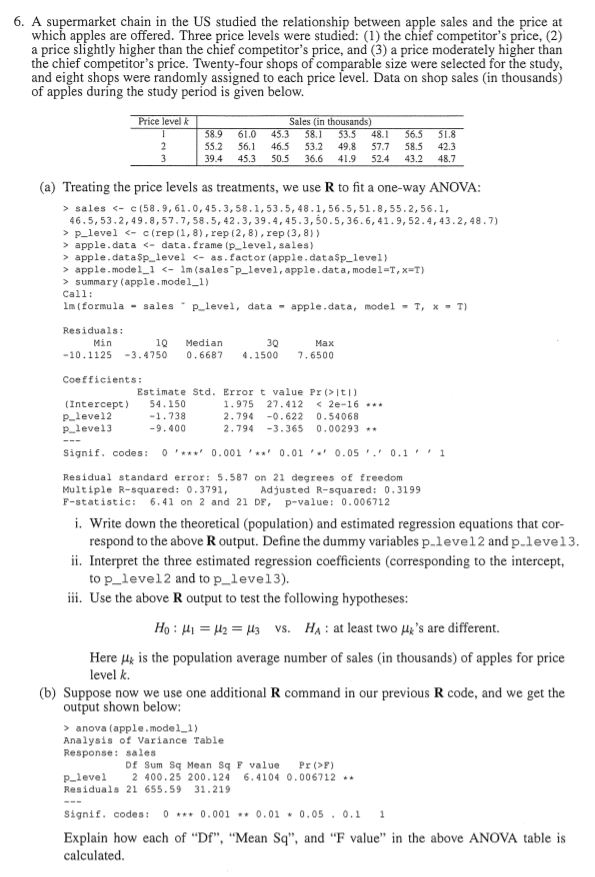Solved: 6. A Supermarket Chain In The US Studied The Relationship Between Apple Sales And The Price At Which Apples Are Offered. Three Price Levels Were Studied: (1) The Chief Competitor's Price, (2)

By |6. A supermarket chain in the US studied the relationship between apple sales and the price at which apples are offered. Three price levels were studied: (1) the chief competitor's price, (2) a price slightly higher than the chief competitor's price, and (3) a price moderately higher than the chief competitor's price. Twenty-four shops of comparable size were selected for the study and eight shops were randomly assigned to each price level. Data on shop sales (in thousands) of apples during the study period is given below. Price level k Sales (in thousands) 58.9 56.5 61.0 56,1 45.3 46.5 50.5 58.1 53.2 53.5 49.8 48.1 51.8 2 55.2 58.5 57.7 42.3 48,7 39.4 45.3 36.6 41,9 52.4 43.2 (a) Treating the price levels as treatments, we use R to fit a one-way ANOVA: sales < e (58.9, 61.0,45.3, 58.1,53.5, 48.1,56.5,51.8,55.2,56.1, 46.5,53.2,49.8,57.7,58.5, 42.3,39.4,45.3,50.5, 36.6,41.9, 52.4,43.2,48.7) > p_level <- c (rep (1, 8), rep (2, 8 ), rep (3, 8)) apple.data apple.dataSp level <as.factor (apple.dataSp level) > apple.model 1 <1m (sales "p_level, apple.data, model-T, x -T ) summary (apple.model_1) <data.frame (p level, sales) Call apple.data, model T, x T m (formula - sales Plevel, data Residuals: Min 10 -3.4750 Median 30 4.1500 ??? 7.6500 -10.1125 0.6687 Coefficients: Estimate Std. Error t value Pr (>Itl) (Intercept) Plevel2 plevel3 54.150 -1.738 -9.400 1.975 2.794 27.412 -0.622 <2e-16 0.54068 0.00293 2.794 -3.365 0 0.001 ' 0.01 0.05 . 0.1 1 Signif, codes: Residual standard error: 5.587 on 21 degrees of freedom Adjusted R-squared: 0.3199 p-value: 0.006712 Multiple R-squared: 0.3791, 6.41 on 2 and 21 DF, F-statistic: i. Write down the theoretical (population) and estimated regression equations that cor- respond to the above R output. Define the dummy variables p.level2 and p.leve13. ii. Interpret the three estimated regression coefficients (corresponding to the intercept, to p level2 and to p level3) i. Use the above R output to test the following hypotheses: HA at least two 's are different. Ho 243 vs. Here is the population average number of sales (in thousands) of apples for price level k. (b) Suppose now we use one additional R command in our previous R code, and we get the output shown below anova (apple.model 1) Analysis of Variance Table Response: sales Df Sum Sq Mean Sq F value 2 400.25 200.124 Pr (>F) 6.4104 0,006712 Plevel Residuals 21 655.59 31.219 0 0.001 Signif. codes: .0.01 0.05.0.1 1 Explain how each of "Df", "Mean Sq", and "F value" in the above ANOVA table is calculated